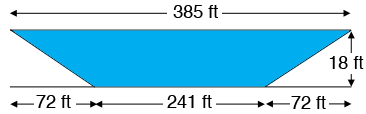SEARCH HOMEMath Central Quandaries & QueriesQuestion from Kelly: I have a pond that measures 335 feet wide, 385 feet long and 18 feet deep with a 4:1 slope on all four side. How do I figure out how many gallons of water this pond will hold?Hi Kelly,

I drew a cross section of the length of your pool (not to scale).$18 \times 4 = 72$ and hence the length of the bottom is $385 - 2\times 72 = 241$ feet. Likewise you can find the width of the bottom and then use the volume calculator at

http://www.aqua-calc.com/calculate/volume-truncated-pyramid

to calculate the volume.

Write back if you need additional help with this,
HarleyMath Central is supported by the University of Regina and The Pacific Institute for the Mathematical Sciences.